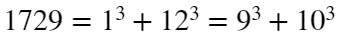# Problem 54089. Ramanujan's Number1729 is the first positive integer which can be expressed as the sum of two positive cubes in two different ways.
Calculate the second, third, fourth ... positive integers which can be expressed as the sum of two positive cubes in two different ways.

### Solution Stats

57.89% Correct | 42.11% Incorrect
Last Solution submitted on Apr 14, 2023

### Community Treasure Hunt

Find the treasures in MATLAB Central and discover how the community can help you!

Start Hunting!